FreeBuf.COM网络安全行业门户，每日发布专业的安全资讯、技术剖析。FreeBuf+小程序

2016-02-16 10:44:28

BOXZ，昵称盒仔，是一款开源的互动娱乐平台！百度一下，有很多盒仔的制作教程，基本都是用arduino制作完成的。假期没事，带着宝宝们用51制作了一个廉价的BOXZ。先上张图：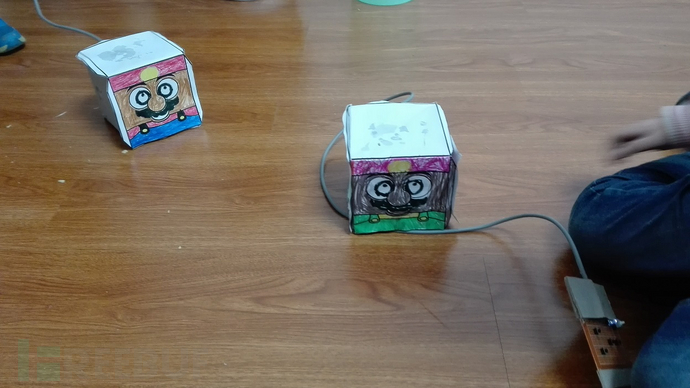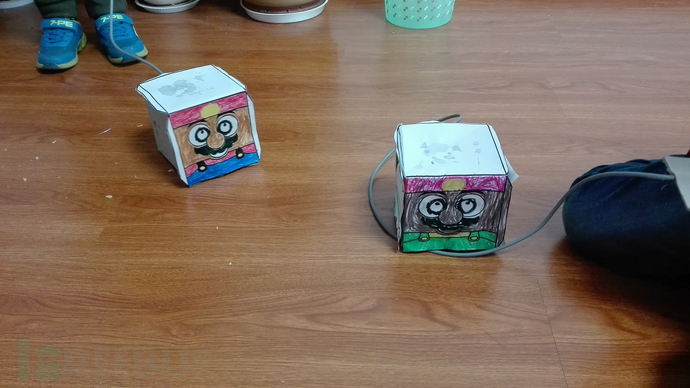1、外壳的制作

（1）下载模板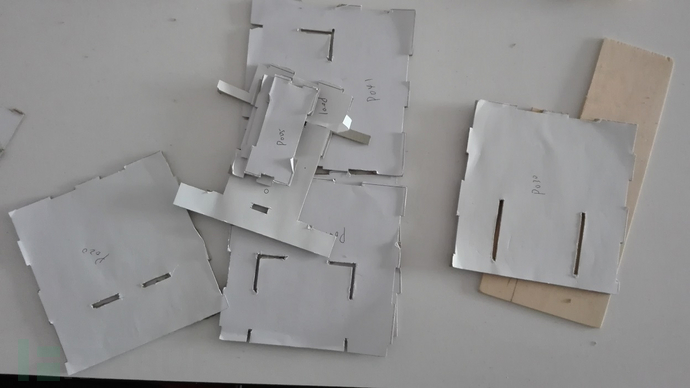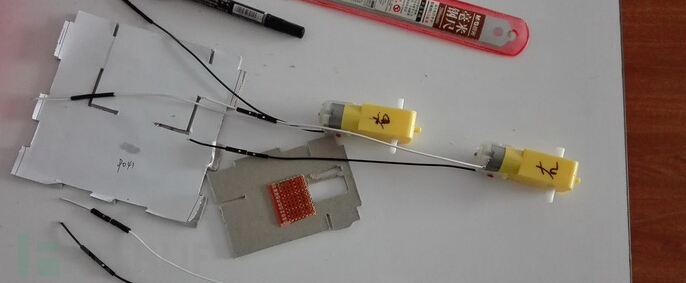（2）组装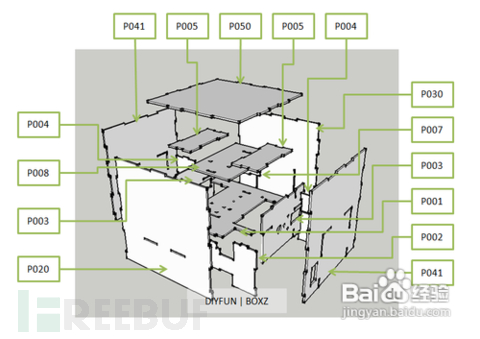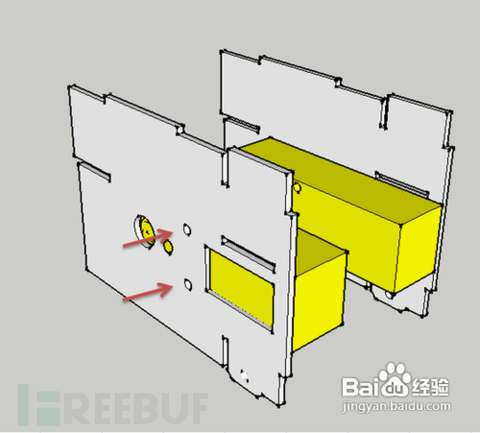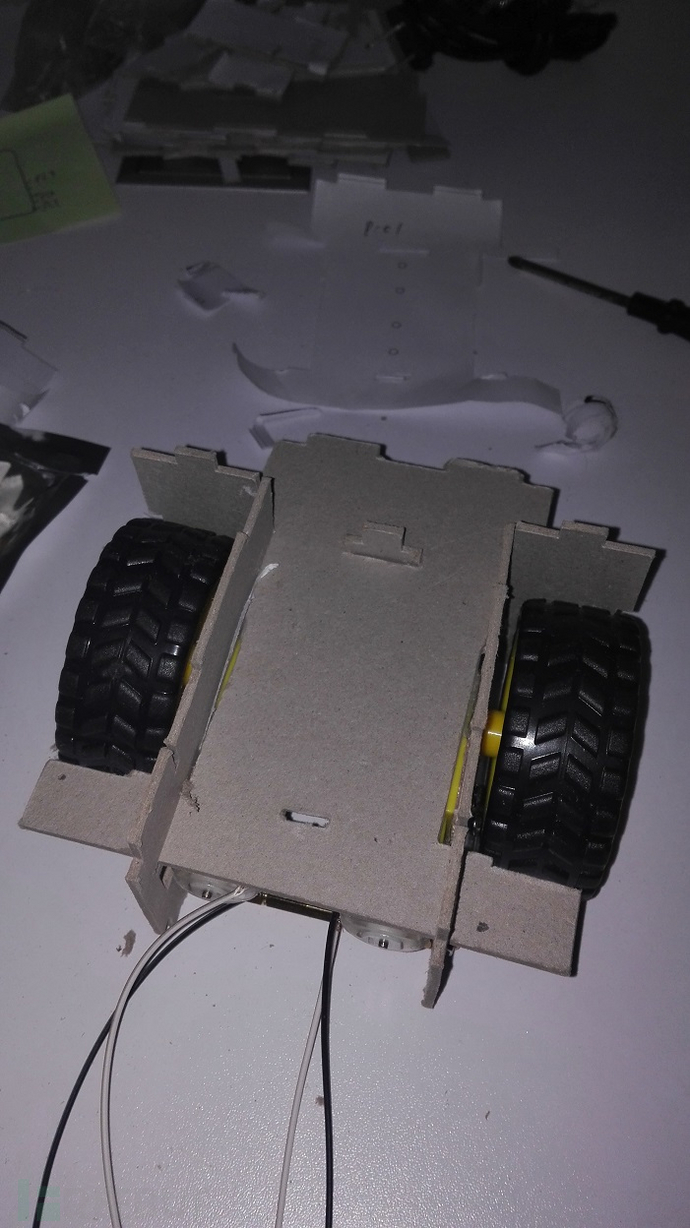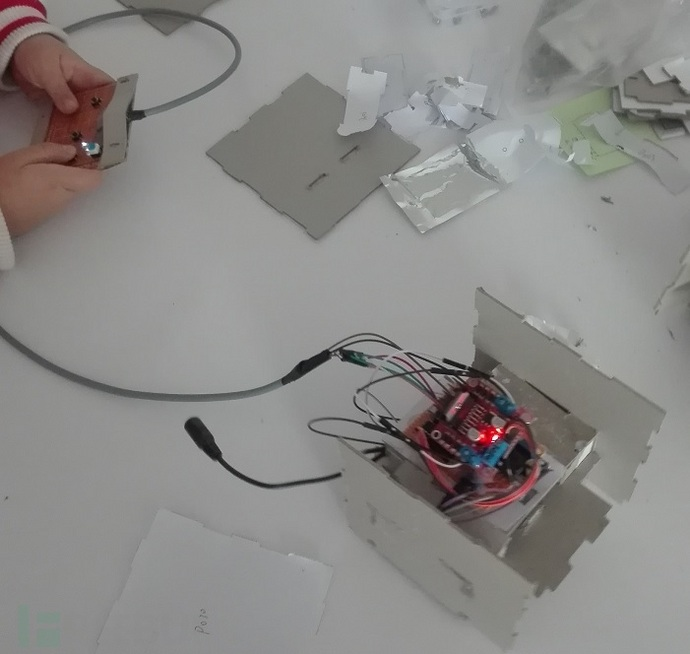2、皮肤的制作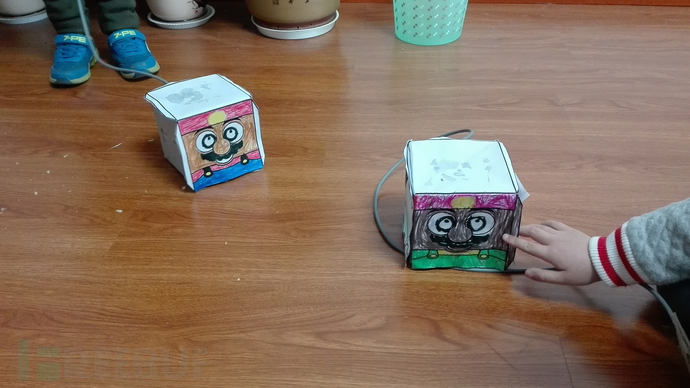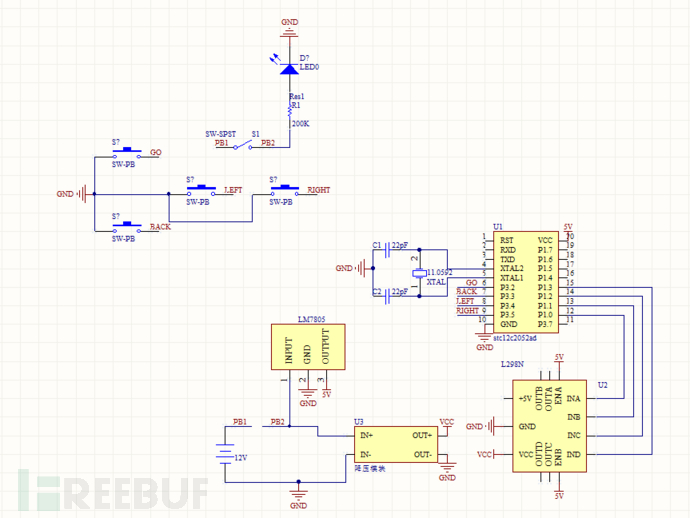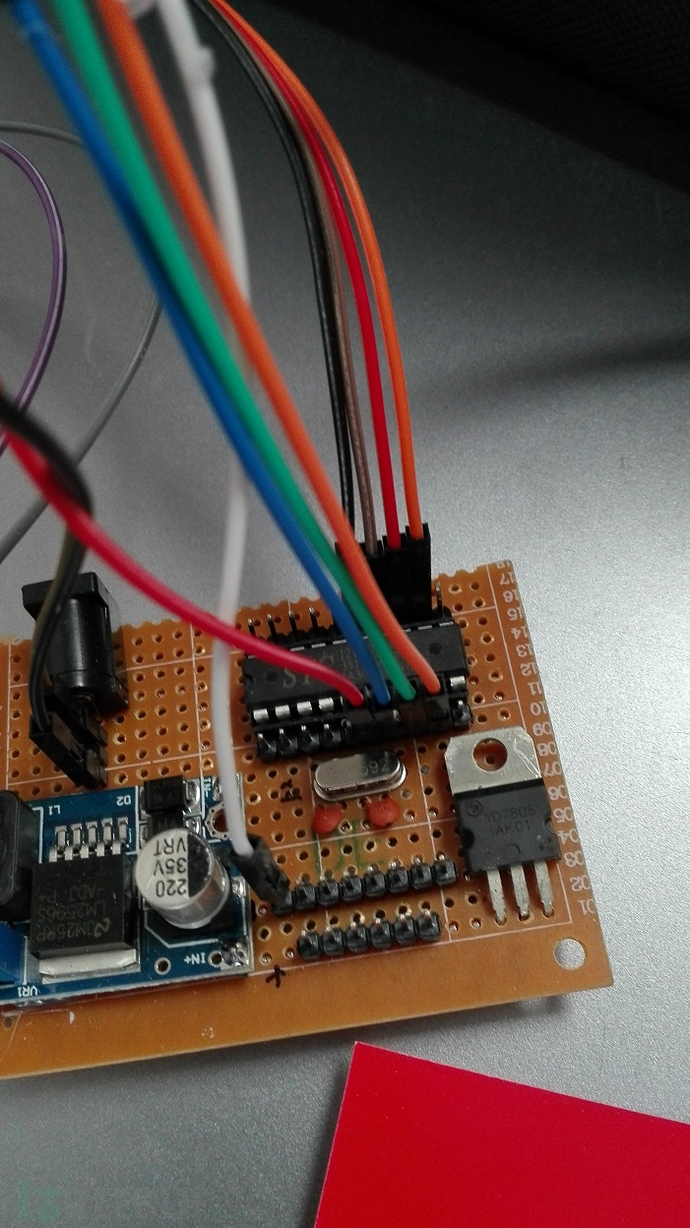``````    #include<stc12c2052ad.h>
#define uint unsigned int
sbit A1=P1^0;// 输出1
sbit A2=P1^1;// 输出2
sbit B1=P1^2;//输出3
sbit B2=P1^3;//输出4
sbit find1=P3^2;//go
sbit find2=P3^3;//back
sbit find3=P3^4;//left
sbit find4=P3^5;//right
void delay_1ms(uint x)
{
uint y,z;
for(y=0;y<x;y++)
for(z=0;z<850;z++) ;
}
void go()
{
A1=0;
A2=1;
B1=0;
B2=1;
}
void back()
{
A1=1;
A2=0;
B1=1;
B2=0;
}
void left()
{
A1=1;
A2=0;
B1=0;
B2=1;
}
void right()
{
A1=0;
A2=1;
B1=1;
B2=0;
}
void find()
{
P3=0xff;
if(find1==0)
go();
if(find2==0)
back();
if(find3==0)
left();
if(find4==0)
right();
if(find1==1&&find2==1&&find3==1&&find4==1)
P1=0x00;
}
void main()
{
while(1)
{
find();
}
}``````

c51的代码如何烧写，这里就不讲了，不会的同学可以自己网上查一下，很简单。

*投稿作者：xiaowuyi，转自须注明来自FreeBuf黑客与极客（FreeBuf.COM）

# 51单片机 # 盒仔机器人

+ 收入我的专辑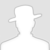• 0 文章数
• 0 评论数
• 0 关注者